Engineering Math - Quick Reference                                 Home : www.sharetechnote.com Green Function   Green Function is a special function which is for a specific operator. It is a function that satisfy the following specific condition. It is named after a British mathematician George Green, who first developed the concept in the 1830s. This is widely used for linear partial differential equation.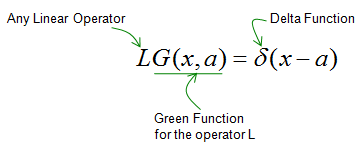Then you may ask 'Why Green Function is important ?', 'Why we have to care about this ?'. It is because this is very useful in some application especially when solving a differential equation.   For example, let's assume that we have a following equation.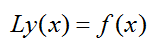If we can derive a Green Function for this operator, you can have the solution for this equation just by applying following equation whatever the y(x) and f(x) is.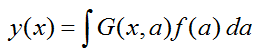Example >   One example for Green Function is as follows. In this case, the operator is '- second derivative'.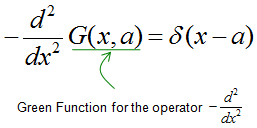A function that can satisfy the above condition is as follows.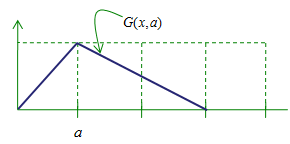How do you know if this function can meet the differential equation (solution for the differential equation). See here.